\$B:#>,\$N0lGU(B

\$BKhF|!":#>,\$N0lGU\$r>e\$2\$F\$\$\$^\$9!#(B
\$B:#>,0{\$`\$b\$N\$K:\$\$C\$?\$i!";29M\$K\$7\$F\$_\$F\$/\$@\$5\$\$!#(B

2022\$BG/(B5\$B7n(B

 1(IJ/K_0%[0=^(B 2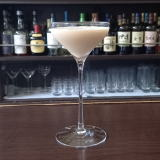(IY<1]%842Y0D^(B 5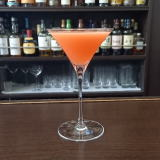(IK_0A%L^[/;Q(B 6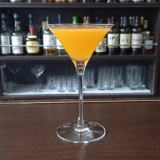(IJ_W@^2=(B 7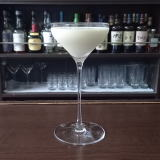(I:^0YC^]%7,C^W/8(B 8 \$B5Y\$_(B 9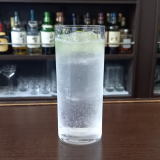(I<^]%DF/8(B 10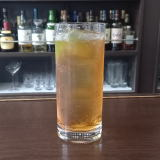(IS=:P-0Y(B 11(IJ^Y0<.](B 12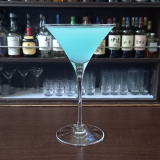M-30(IZ2](B 13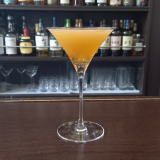(I=2=%L'PX0(B 14 \$B5Y\$_(B 15 \$B5Y\$_(B 16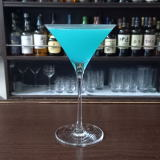(IL^Y0%DX/L_(B 17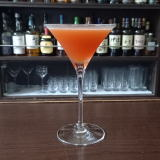(I<,]J2(B 18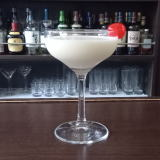(I80Y%J^EE(B 19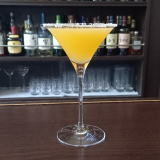(IZ/D^%W25](B 20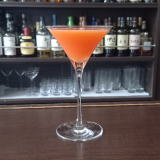(IL[X@^(B 21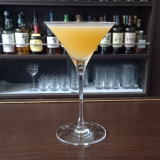(I;2D^60(B 22 \$B5Y\$_(B 23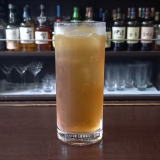(IOP0%C2W0(B 24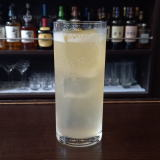(I<^]%L(=^(B 25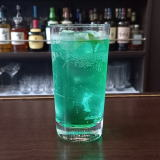(I[]8^%8^X0](B 26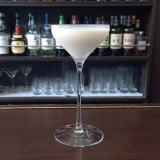(I[0D^W]E0(B 27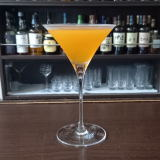(I17@^8D(B 28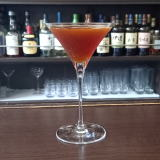(IO3]D%L<^(B 29 \$B5Y\$_(B 30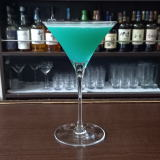(I=62%1]D^%<0(B 31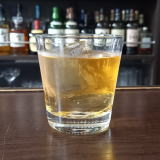(IW=C(%H2Y(B